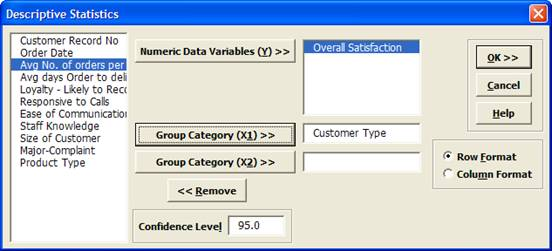Include Top

# How Do I Calculate Confidence Intervals in Excel Using SigmaXL?

1. Open Customer Data.xlsx. Click Sheet 1 Tab (or press F4 to activate last worksheet).

2. Click SigmaXL > Statistical Tools > Descriptive Statistics.

3. Check Use Entire Data Table, click Next.

4. Select Overall Satisfaction, click Numeric Data Variables (Y) >>. Select Customer Type, click Group Category (X1) >>; Confidence Level default is 95%:

5.6. Click OK. Descriptive Statistics are given for Customer Satisfaction grouped by Customer Type:

7.We are given the 95% confidence interval for each sample Mean (95% CI Mean) as well as the 95% confidence interval for the Standard Deviation (95% CI Sigma – do not confuse this with Process Sigma Quality Level).

These confidence intervals are very important in understanding our data and making decisions from the data. How often are we driven by sample estimates only and fail to consider the confidence interval or margin of error? For example, newspapers will often fail to take into account the confidence interval when reporting opinion poll results. (To calculate confidence intervals for discrete proportion data, use SigmaXL > Templates and Calculators > Basic Statistical Templates > 1 Proportion Confidence Interval).

Note that a confidence interval of 95% implies that, on average, the true population parameter (Mean, Median, Standard Deviation, or Proportion) will lie within the interval 19 times out of 20.

A confidence interval or margin of error does not take into account measurement error or survey bias, so the actual uncertainty may be greater than stated. This should be addressed with good data collection, reliable measurement systems, and good survey design.

Confidence Intervals for the Mean can be obtained in several ways with SigmaXL: Descriptive Statistics, Histograms & Descriptive Statistics, 1-Sample t-test & Confidence Intervals, One-Way ANOVA, and Multi-Vari Charts.

To illustrate confidence intervals for the mean of Overall Satisfaction graphically, we have generated a Multi-Vari Chart (with 95% CI Mean Options) using the Customer Data.xls data. This chart type is covered here.The dots correspond to individual data points. The tick marks show the 95% upper confidence limit, mean, and 95% lower confidence limit. Clearly we can see that Customer Type 2 has a significantly higher level of mean satisfaction; the lower limit does not overlap with the upper limit for Types 1 and 3. On the other hand, we see overlap of the CI’s when comparing types 1 and 3. Hypothesis testing will now be used to compare the mean satisfaction scores more precisely and determine statistical significance for the results.

# Web Demos

Our CTO and Co-Founder, John Noguera, regularly hosts free Web Demos featuring SigmaXL and DiscoverSim